Combining Inferences from Imputed Data Sets

Withimputations,different sets of the point and variance estimates for a parameter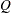can be computed. Suppose that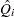and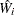are the point and variance estimates, respectively, from theth imputed data set,= 1, 2, ...,. Then the combined point estimate forfrom multiple imputation is the average of thecomplete-data estimates: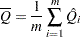Suppose that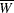is the within-imputation variance, which is the average of thecomplete-data estimates: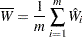And suppose that
is the between-imputation variance: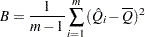Then the variance estimate associated with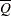is the total variance (Rubin 1987)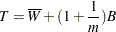The statistic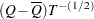is approximately distributed aswith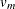degrees of freedom (Rubin 1987), where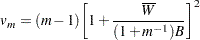The degrees of freedomdepend onand the ratio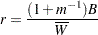The ratiois called the relative increase in variance due to nonresponse (Rubin 1987). When there is no missing information about, the values ofandare both zero. With a large value ofor a small value of, the degrees of freedomwill be large and the distribution ofwill be approximately normal.

Another useful statistic is the fraction of missing information about: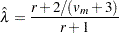Both statisticsand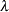are helpful diagnostics for assessing how the missing data contribute to the uncertainty about.

When the complete-data degrees of freedom
are small, and there is only a modest proportion of missing data, the computed degrees of freedom,, can be much larger than, which is inappropriate. For example, with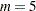and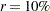, the computed degrees of freedom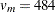, which is inappropriate for data sets with complete-data degrees of freedom less than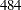.

Barnard and Rubin (1999) recommend the use of adjusted degrees of freedom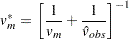where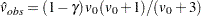and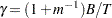.

If you specify the complete-data degrees of freedomwith the EDF= option, the MIANALYZE procedure uses the adjusted degrees of freedom,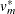, for inference. Otherwise, the degrees of freedomare used.